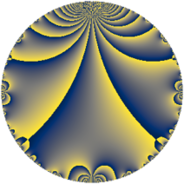# Properties

 Label 5.4.aLevel $5$ Weight $4$ Character orbit 5.a Rep. character $\chi_{5}(1,\cdot)$ Character field $\Q$ Dimension $1$ Newform subspaces $1$ Sturm bound $2$ Trace bound $0$

# Related objects

## Defining parameters

 Level: $$N$$ $$=$$ $$5$$ Weight: $$k$$ $$=$$ $$4$$ Character orbit: $$[\chi]$$ $$=$$ 5.a (trivial) Character field: $$\Q$$ Newform subspaces: $$1$$ Sturm bound: $$2$$ Trace bound: $$0$$

## Dimensions

The following table gives the dimensions of various subspaces of $$M_{4}(\Gamma_0(5))$$.

Total New Old
Modular forms 3 1 2
Cusp forms 1 1 0
Eisenstein series 2 0 2

The following table gives the dimensions of the cuspidal new subspaces with specified eigenvalues for the Atkin-Lehner operators and the Fricke involution.

$$5$$Dim.
$$+$$$$1$$

## Trace form

 $$q - 4 q^{2} + 2 q^{3} + 8 q^{4} - 5 q^{5} - 8 q^{6} + 6 q^{7} - 23 q^{9} + O(q^{10})$$ $$q - 4 q^{2} + 2 q^{3} + 8 q^{4} - 5 q^{5} - 8 q^{6} + 6 q^{7} - 23 q^{9} + 20 q^{10} + 32 q^{11} + 16 q^{12} - 38 q^{13} - 24 q^{14} - 10 q^{15} - 64 q^{16} + 26 q^{17} + 92 q^{18} + 100 q^{19} - 40 q^{20} + 12 q^{21} - 128 q^{22} - 78 q^{23} + 25 q^{25} + 152 q^{26} - 100 q^{27} + 48 q^{28} - 50 q^{29} + 40 q^{30} - 108 q^{31} + 256 q^{32} + 64 q^{33} - 104 q^{34} - 30 q^{35} - 184 q^{36} + 266 q^{37} - 400 q^{38} - 76 q^{39} + 22 q^{41} - 48 q^{42} + 442 q^{43} + 256 q^{44} + 115 q^{45} + 312 q^{46} - 514 q^{47} - 128 q^{48} - 307 q^{49} - 100 q^{50} + 52 q^{51} - 304 q^{52} + 2 q^{53} + 400 q^{54} - 160 q^{55} + 200 q^{57} + 200 q^{58} + 500 q^{59} - 80 q^{60} - 518 q^{61} + 432 q^{62} - 138 q^{63} - 512 q^{64} + 190 q^{65} - 256 q^{66} + 126 q^{67} + 208 q^{68} - 156 q^{69} + 120 q^{70} + 412 q^{71} - 878 q^{73} - 1064 q^{74} + 50 q^{75} + 800 q^{76} + 192 q^{77} + 304 q^{78} + 600 q^{79} + 320 q^{80} + 421 q^{81} - 88 q^{82} + 282 q^{83} + 96 q^{84} - 130 q^{85} - 1768 q^{86} - 100 q^{87} - 150 q^{89} - 460 q^{90} - 228 q^{91} - 624 q^{92} - 216 q^{93} + 2056 q^{94} - 500 q^{95} + 512 q^{96} + 386 q^{97} + 1228 q^{98} - 736 q^{99} + O(q^{100})$$

## Decomposition of $$S_{4}^{\mathrm{new}}(\Gamma_0(5))$$ into newform subspaces

Label Dim $A$ Field CM Traces A-L signs $q$-expansion
$a_{2}$ $a_{3}$ $a_{5}$ $a_{7}$ 5
5.4.a.a $1$ $0.295$ $$\Q$$ None $$-4$$ $$2$$ $$-5$$ $$6$$ $+$ $$q-4q^{2}+2q^{3}+8q^{4}-5q^{5}-8q^{6}+\cdots$$